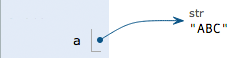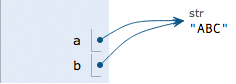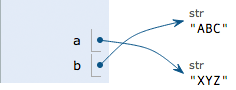# 数据类型和变量

### 数据类型

#### 整数

Python可以处理任意大小的整数，当然包括负整数，在程序中的表示方法和数学上的写法一模一样，例如：`1``100``-8080``0`，等等。

#### 字符串

``````'I\'m \"OK\"!'
``````

``````I'm "OK"!
``````

``````>>> print 'I\'m ok.'
I'm ok.
>>> print 'I\'m learning\nPython.'
I'm learning
Python.
>>> print '\\\n\\'
\
\
``````

``````>>> print '\\\t\\'
\       \
>>> print r'\\\t\\'
\\\t\\
``````

``````>>> print '''line1
... line2
... line3'''
line1
line2
line3
``````

``````print '''line1
line2
line3'''
``````

#### 布尔值

``````>>> True
True
>>> False
False
>>> 3 > 2
True
>>> 3 > 5
False
``````

`and`运算是与运算，只有所有都为`True``and`运算结果才是`True`

``````>>> True and True
True
>>> True and False
False
>>> False and False
False
``````

`or`运算是或运算，只要其中有一个为`True``or`运算结果就是`True`

``````>>> True or True
True
>>> True or False
True
>>> False or False
False
``````

`not`运算是非运算，它是一个单目运算符，把`True`变成`False``False`变成`True`

``````>>> not True
False
>>> not False
True
``````

``````if age >= 18:
else:
print 'teenager'
``````

### 变量

``````a = 1
``````

``````t_007 = 'T007'
``````

``````Answer = True
``````

``````a = 123 # a是整数
print a
a = 'ABC' # a变为字符串
print a
``````

``````int a = 123; // a是整数类型变量
a = "ABC"; // 错误：不能把字符串赋给整型变量
``````

``````x = 10
x = x + 2
``````

``````a = 'ABC'
``````

1. 在内存中创建了一个`'ABC'`的字符串；

2. 在内存中创建了一个名为`a`的变量，并把它指向`'ABC'`

``````a = 'ABC'
b = a
a = 'XYZ'
print b
``````### 常量

``````PI = 3.14159265359
``````

``````>>> 10 / 3
3
``````

``````>>> 10.0 / 3
3.3333333333333335
``````

``````>>> 10 % 3
1
``````

### 小结

Python支持多种数据类型，在计算机内部，可以把任何数据都看成一个“对象”，而变量就是在程序中用来指向这些数据对象的，对变量赋值就是把数据和变量给关联起来。